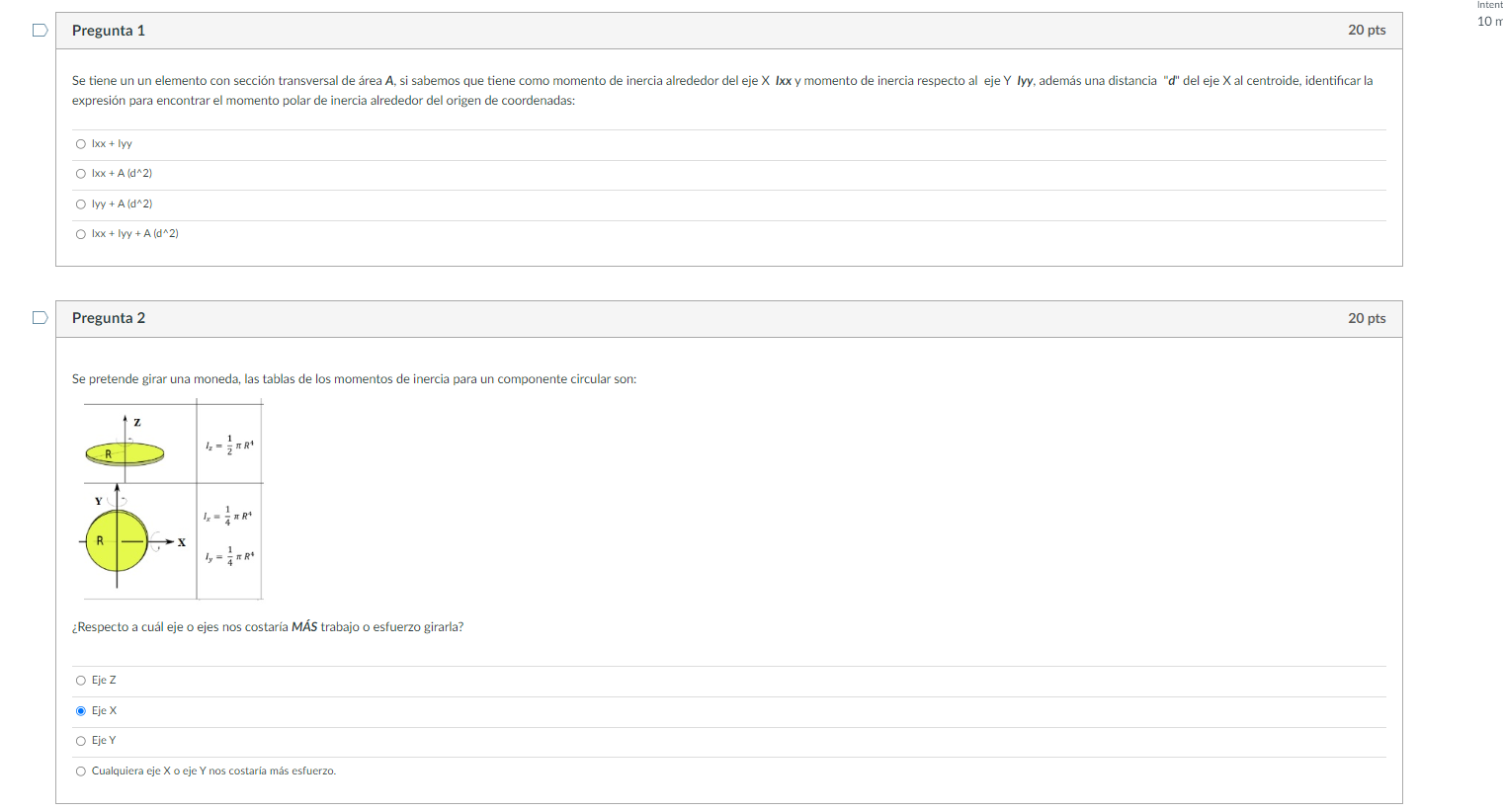# Question ​​​​​​​ expresión para encontrar el momento polar de inercia alrededor del origen de coordenadas: \begin{tabular}{l} $$1 x x+\mid y y$$ \\ \hline $$\mid x x+A\left(d^{\wedge} 2\right)$$ \\ \hline $$\mid y y+A\left(d^{\wedge} 2\right)$$ \\ \hline$$|x x+| y y+A\left(d^{\wedge} 2\right)$$ \end{tabular} Pregunta 2 Se pretende girar una moneda, las tablas de los momentos de inercia para un componente circular son: ¿Respecto a cuál eje o ejes nos costaria MÁS trabajo o esfuerzo girarla? Eje $$Z$$ Eje X Eje $$Y$$ Cualquiera eje $$X$$ o eje $$Y$$ nos costaría más esfuerzo.9SSQMI The Asker · Mechanical Engineering​​​​​​​

Transcribed Image Text: expresión para encontrar el momento polar de inercia alrededor del origen de coordenadas: \begin{tabular}{l} $$1 x x+\mid y y$$ \\ \hline $$\mid x x+A\left(d^{\wedge} 2\right)$$ \\ \hline $$\mid y y+A\left(d^{\wedge} 2\right)$$ \\ \hline$$|x x+| y y+A\left(d^{\wedge} 2\right)$$ \end{tabular} Pregunta 2 Se pretende girar una moneda, las tablas de los momentos de inercia para un componente circular son: ¿Respecto a cuál eje o ejes nos costaria MÁS trabajo o esfuerzo girarla? Eje $$Z$$ Eje X Eje $$Y$$ Cualquiera eje $$X$$ o eje $$Y$$ nos costaría más esfuerzo.
More
Transcribed Image Text: expresión para encontrar el momento polar de inercia alrededor del origen de coordenadas: \begin{tabular}{l} $$1 x x+\mid y y$$ \\ \hline $$\mid x x+A\left(d^{\wedge} 2\right)$$ \\ \hline $$\mid y y+A\left(d^{\wedge} 2\right)$$ \\ \hline$$|x x+| y y+A\left(d^{\wedge} 2\right)$$ \end{tabular} Pregunta 2 Se pretende girar una moneda, las tablas de los momentos de inercia para un componente circular son: ¿Respecto a cuál eje o ejes nos costaria MÁS trabajo o esfuerzo girarla? Eje $$Z$$ Eje X Eje $$Y$$ Cualquiera eje $$X$$ o eje $$Y$$ nos costaría más esfuerzo.&#12304;General guidance&#12305;The answer provided below has been developed in a clear step by step manner.Step1/21. The area moment of inertia of the area about x axis and y axis given as $$\mathrm{{I}_{{{x}{x}}}}$$ and $$\mathrm{{I}_{{{y}{y}}}}$$ . The x and y axis cross at origin, perpendicularly. So the polar moment of inertia of the area about origin is \begin{align*} \mathrm{{J}_{\text{origin}}} &= \mathrm{{I}_{{{x}{x}}}+{I}_{{{y}{y}}}} \end{align*}So the correct option is $$\mathrm{\text{(1st option)}\ \ {I}_{{{x}{x}}}+{I}_{{{y}{y}}}}$$ ExplanationSince the polar moment of inertia is asked about the interesting point of x and y axis, we don't need to shift the given area moment axis. Explanation:Please refer to solution in this step.Step2/22. The power required can be expressed as\( \begin{align*} \mathrm{{P}} &= \mathrm{\text{Torque (T)}\time ... See the full answer